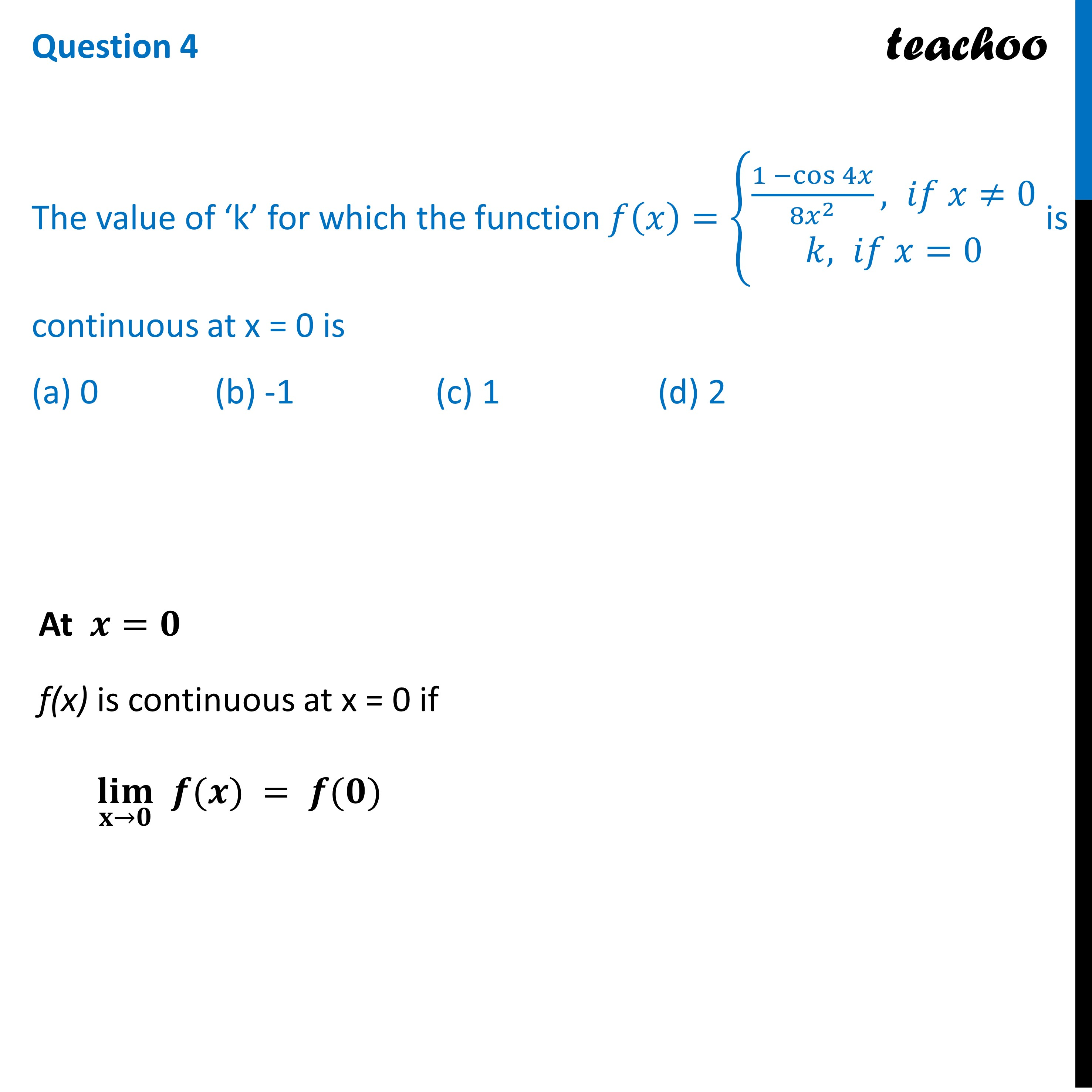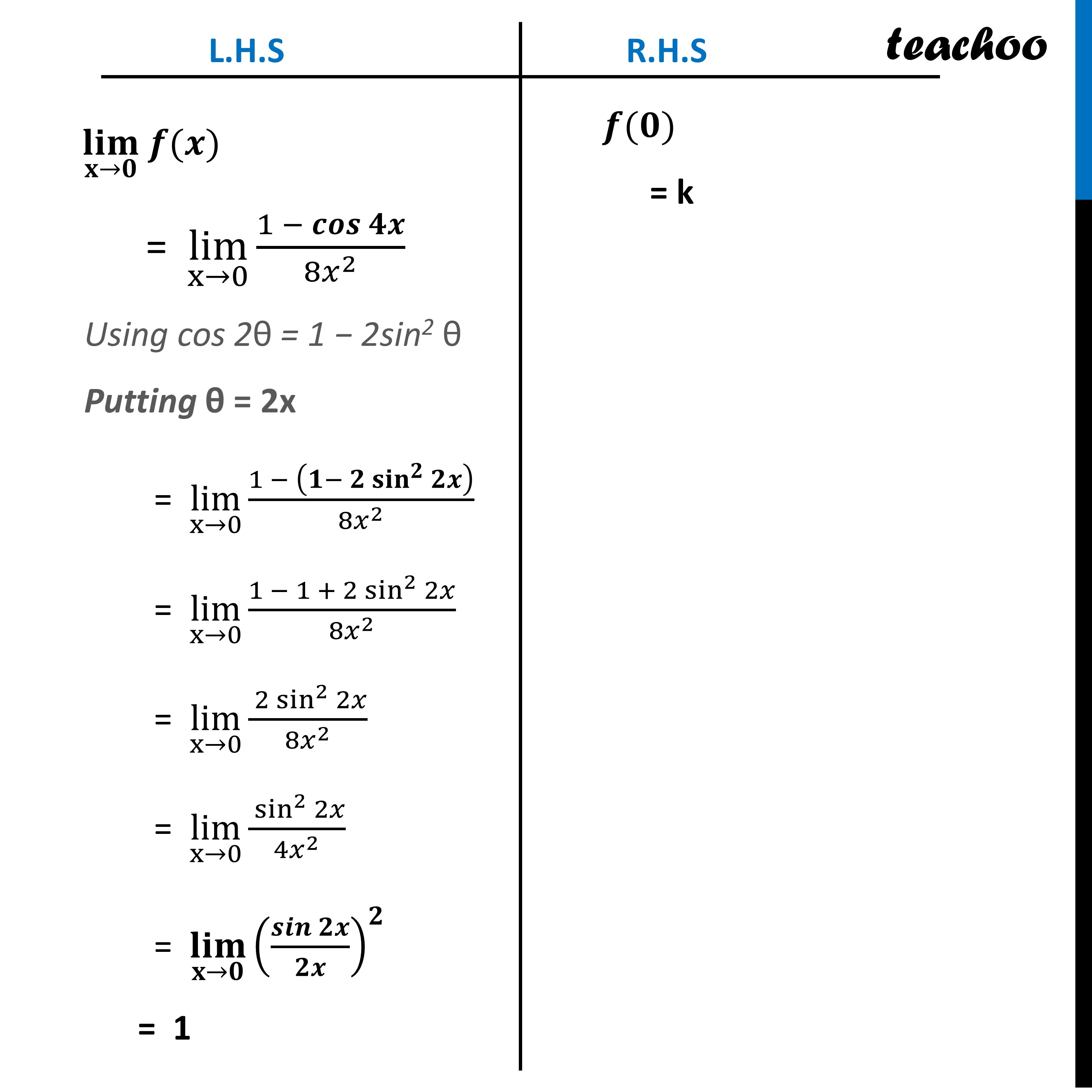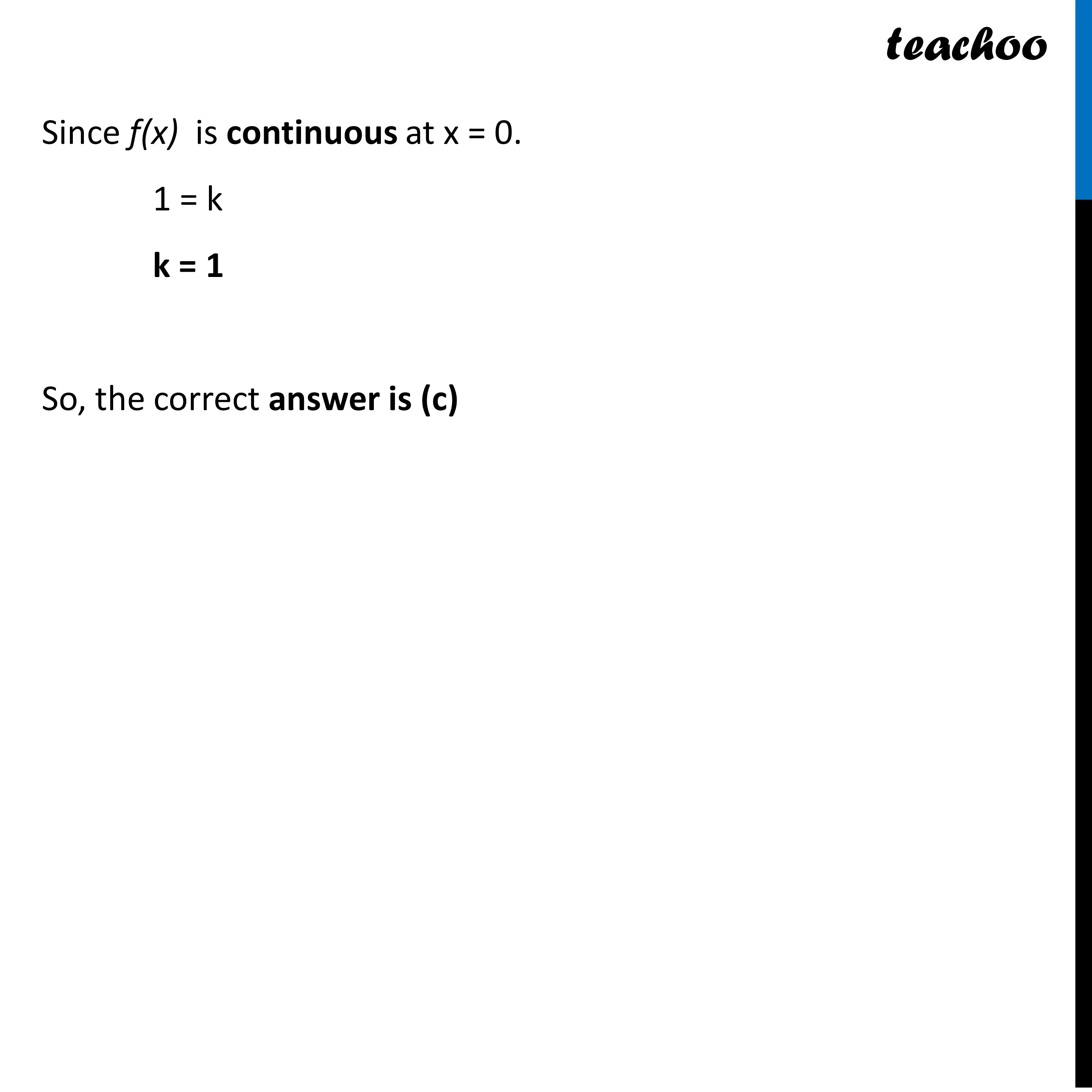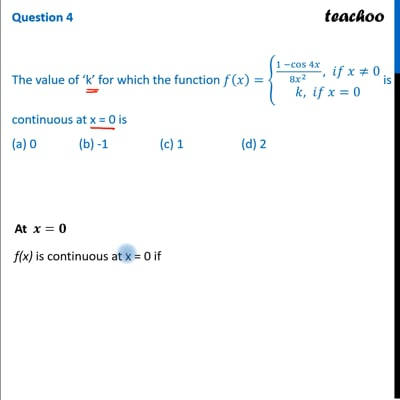CBSE Class 12 Sample Paper for 2023 Boards

Class 12
Solutions of Sample Papers and Past Year Papers - for Class 12 Boards

## (a) 0              (b) -1                 (c) 1                   (d) 2This video is only available for Teachoo black users

Learn in your speed, with individual attention - Teachoo Maths 1-on-1 Class

### Transcript

Question 4 The value of ‘k’ for which the function 𝑓(𝑥)={█((1 −cos⁡4𝑥)/〖8𝑥〗^2 , 𝑖𝑓 𝑥≠0@&𝑘, 𝑖𝑓 𝑥=0)┤ is continuous at x = 0 is (a) 0 (b) -1 (c) 1 (d) 2At 𝒙=𝟎 f(x) is continuous at x = 0 if (𝐥𝐢𝐦)┬(𝐱→𝟎) 𝒇(𝒙) = 𝒇(𝟎) L.H.S (𝐥𝐢𝐦)┬(𝐱→𝟎) 𝒇(𝒙) "= " lim┬(x→0) (1 − 𝒄𝒐𝒔⁡𝟒𝒙)/〖8𝑥〗^2 Using cos 2θ = 1 − 2sin2 θ Putting θ = 2x "= " lim┬(x→0) (1 − (𝟏− 𝟐 〖𝐬𝐢𝐧〗^𝟐⁡𝟐𝒙 ))/〖8𝑥〗^2 "= " lim┬(x→0) (1 − 1 + 2 sin^2⁡2𝑥)/〖8𝑥〗^2 "= " lim┬(x→0) ( 2 sin^2⁡2𝑥)/〖8𝑥〗^2 "= " lim┬(x→0) ( sin^2⁡2𝑥)/〖4𝑥〗^2 "= " (𝐥𝐢𝐦)┬(𝐱→𝟎) (𝒔𝒊𝒏⁡𝟐𝒙/𝟐𝒙)^𝟐 = 1 R.H.S 𝒇(𝟎) = k Since f(x) is continuous at x = 0. 1 = k k = 1 So, the correct answer is (c)